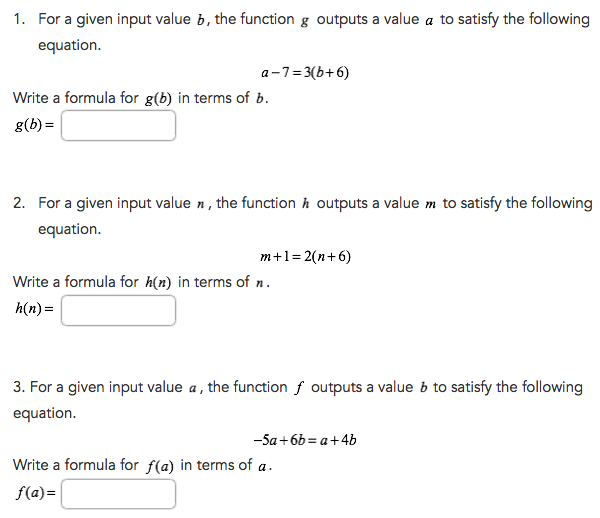Obtaining a Function From an Equation
starstarstarstarstarstarstarstarstarstar
by Brandon Seay
| 11 Questions
Note from the author:
Writing a function from an equation.

# Difference between Equations and Functions

Key difference: In mathematics, an equation is used to denote the equality between two expressions. A function, on the other hand, is in much more complex than an equation. A function is used to denote a relation between a set of inputs and a set of corresponding outputs.
An equation and a function are two of the basic foundations of algebra, a subject matter under mathematics. Both can be quite complex in nature, however, in their basic form they may be confused as each other. The main difference between an equation and a function is the fact that an equation usually deals with a single input, whereas, a function can have numerous inputs.

In mathematics, an equation is used to denote the equality between two expressions. Essentially, an equation is written as an expression equals to another expression. For example: x + 2 = 5. This denotes that whatever x is, if you add 2 to it, will be equal to 5. Hence, we can solve the equation for x, which is 3, as 3 + 2 = 5.
Equations can be more complex than that and can include more than one variables, such as x, y, z, etc. in a single equation. For example: 3x + 2y – z = 4. However, each variable will correspond to one number. In this case, x = 1, y = 2 and z = 3.
Hence,
3x + 2y – z = 4
becomes 3(1) + 2(2) – 3 = 4
which is 3 + 4 – 3 = 4
essentially 4 = 4

A function, on the other hand, is in much more complex than an equation. A function is used to denote a relation between a set of inputs and a set of corresponding outputs. Essentially, an input should give a single output. A function is a relationship between two variables. For example: f(x) = x + 2. As per this function, whatever, the input is, it will give you a single output, which will be the input plus 2. Let’s solve this function:1
2
3
4
5
1
1
2
1
3
1
4
1
5
1
And so on…
Functions have various applications in mathematics, physics, computer science, etc. They can range from a simple function, as denoted above, to complex formulas or algorithms. A function can be thought of as a machine that gives an output for an input, and that output is somehow related to the input.
A function always has three parts: the input, the relationship, and the output. The classic way of writing a function is with "f(x) = ... ", where x denotes the input and f(x) denotes the output.6
7
8
6
1
7
1
8
1

# Obtaining a function from an Equation

HSF.IF.A.1
Objective: Rewrite an equation in terms of a variable to represent a function.

Equations and functions are not the same thing, but they can be related in several ways. In this lesson, we obtain a function from an equation. The function represents the same relationship between the quantities as the equation.

# Example #1

For a given input value q, the function g outputs a value r to satisfy the following equation:
Write a formula for g(q) in terms of q.
Rewrite the equation by solving for r. (in terms of q means we are solving for r)
1. Add 7q to each side.
2. Add 4r to each side.
3. Divide each side by 16.
4. Rewrite in function notation by replacing r with g(q).

# Example #2

For a given input value b, the function g outputs a value a to satisfy the following equation.
a-7=3(b+2)
Write a formula for g(b), in terms of b.
Rewrite the equation by solving for a. (in terms of b means we are solving for a)
1. Distribute 3 into b+2
2. Add 7 to each side.
3. Rewrite in function notation by replacing a with g(b)9
10
11
9
1
10
1
11
1